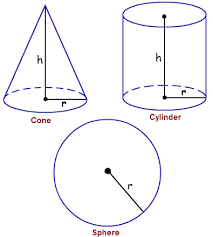# Volume comparison!

Geometry Level 2Which of the following shapes has a greater volume?

a) A cone of height $r$ and radius of base $r$
b) A cylinder of base radius $r$ and height $r$
c) A hemisphere of radius $r$

Note: The measurement in the picture is not included in the problem.

×